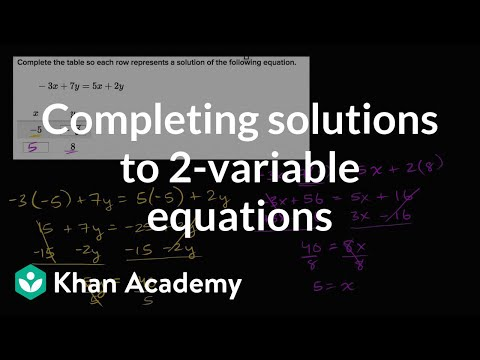Video

# Systems of equations with substitution: -3x - 4y = -2 and y = 2x - 5 (Full video)

Khan Academy

## Supporting grades: 8

Description: Sal demonstrates how to solve the system of equations -3x - 4y = -2 and y = 2x - 5 using the substitution method. So that it's less likely that we get shown up by talking birds in the future, we've set a little bit of exercise for solving systems of equations with substitution. So, what's neat about this is that they've already solved the second equation. We can take this constraint, the constraint on y in terms of x and substitute it for y in this first blue equation and then solve for x.

You must log inorsign upif you want to:*

*Teacher Advisor is 100% free.

### Other videos you might be interested in### Solving Systems of Equations by Substitution (Full video)

#### Student Achievement Partners